NEET  >  Kinematic Equations for Uniformly Accelerated Motion

# Kinematic Equations for Uniformly Accelerated Motion - Notes | Study Physics Class 11 - NEET

 Table of contentsKinematic Equations of MotionDerivation of Equation of Motion1 Crore+ students have signed up on EduRev. Have you?

Kinematic Equations of Motion

If an object starts with velocity ”u” and after some time “t” its velocity changes to v, if the uniform acceleration is a and distance traveled in time (t) is s, then we obtain the following kinematic equations of uniformly accelerated motion.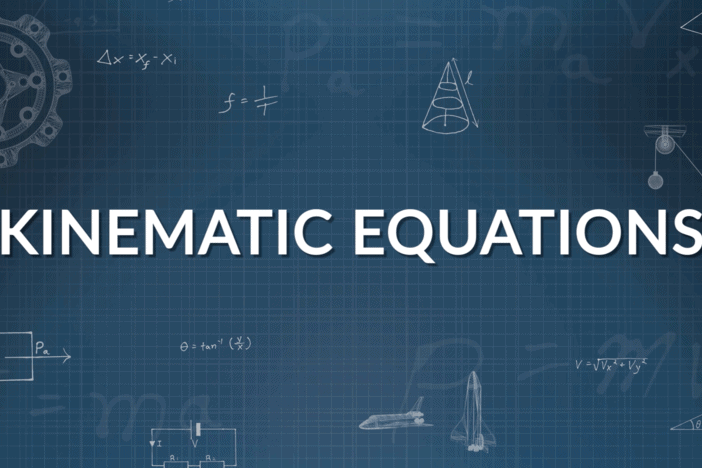Equations of motion of kinematics describe the basic concept of the motion of an object such as the position, velocity, or the acceleration of an object at various times. These three equations of motion govern the motion of an object in 1D, 2D and 3D. The derivation of the equations of motion is one of the most important topics in Physics. In this article, we will show you how to derive the first, second and third equation of motion by graphical method, algebraic method and calculus method.

Definition of Equations of Motion

Equations of motion, in physics, are defined as equations that describe the behaviour of a physical system in terms of its motion as a function of time.

There are three equations of motion that can be used to derive components such as displacement(s), velocity (initial and final), time(t) and acceleration(a). The following are the three equation of motion:

• First Equation of Motion: v = u + at
• Second Equation of Motion: s = ut + 1/2at2
• Third Equation of Motion: v= u+ 2as

Derivation of Equation of Motion

The equations of motion can be derived using the following methods:

• Derivation of equations of motion by Simple Algebraic Method
• Derivation of equations of Motion by Graphical Method
• Derivation equations of Motion by Calculus Method

In the next few sections, the equations of motion are derived by all the three methods in a simple and easy to understand way.

Derivation of First Equation of Motion

For the derivation, let us consider a body moving in a straight line with uniform acceleration. Then, let the initial velocity be u, acceleration is denoted as a, time period is denoted as t, velocity is denoted as v, and the distance travelled is denoted as s.

Derivation of First Equation of Motion by Algebraic Method

We know that the acceleration of the body is defined as the rate of change of velocity.

Mathematically, acceleration is represented as follows: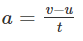where v is the final velocity and u is the initial velocity.
Rearranging the above equation, we arrive at the first equation of motion as follows:

v = u + at

Derivation of First Equation of Motion by Graphical Method

The first equation of motion can be derived using a velocity-time graph for a moving object with an initial velocity of u, final velocity v, and acceleration a.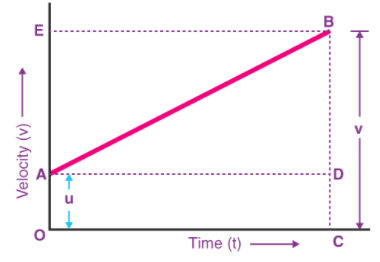In the above graph,

• The velocity of the body changes from A to B in time t at a uniform rate.
• BC is the final velocity and OC is the total time t.
• A perpendicular is drawn from B to OC, a parallel line is drawn from A to D, and another perpendicular is drawn from B to OE (represented by dotted lines).

Following details are obtained from the graph above:
The initial velocity of the body, u = OA
The final velocity of the body, v = BC
From the graph, we know that
BC = BD + DC
Therefore, v = BD + DC
v = BD + OA (since DC = OA)
Finally,
v = BD + u (since OA = u) (Equation 1)
Now, since the slope of a velocity-time graph is equal to acceleration a,
So,
a = slope of line AB
Since AD = AC = t, the above equation becomes:
BD = at (Equation 2)
Now, combining Equation 1 & 2, the following is obtained:
v = u + at
Derivation of First Equation of Motion by Calculus Method
Since acceleration is the rate of change of velocity, it can be mathematically written as:
a = dv/dt
Rearranging the above equation, we get
Integrating both the sides, we get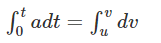at = v − u

Rearranging, we get

v = u + at

Derivation of Second Equation of Motion

For the derivation of the second equation of motion, consider the same variables that were used for derivation of the first equation of motion.

Derivation of Second Equation of Motion by Algebraic Method

Velocity is defined as the rate of change of displacement. This is mathematically represented as:

Velocity = Displacement/Time
Rearranging, we get
Displacement = Velcoity × Time
If the velocity is not constant then in the above equation we can use average velocity in the place of velocity and rewrite the equation as follows:
Displacement =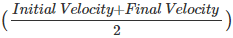× Time

Substituting the above equations with the notations used in the derivation of the first equation of motion, we get
s = u+v/2 × t

From the first equation of motion, we know that v = u + at. Putting this value of v in the above equation, we get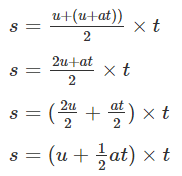On further simplification, the equation becomes:
s = ut + 1/2 at2

Derivation of Second Equation of Motion by Graphical Method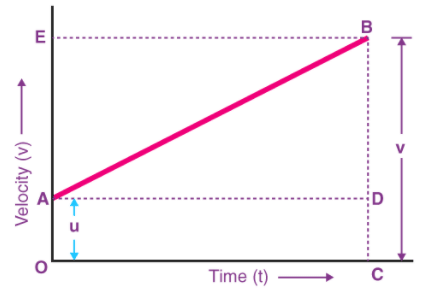From the graph above, we can say that
Distance travelled (s) = Area of figure OABC = Area of rectangle OADC + Area of triangle ABD
s = (1/2AB × BD)+(OA × OC)
Since BD = EA, the above equation becomes
s = (1/2AB × EA) + (u × t)

As EA = at, the equation becomes
s = 1/2 × at × t + ut

On further simplification, the equation becomes
s = ut + 1/2at2

Derivation of Second Equation of Motion by Calculus Method

Velocity is the rate of change of displacement.

Mathematically, this is expressed as

v = ds/dt
Rearranging the equation, we get
ds = vdt
Substituting the first equation of motion in the above equation, we get
ds = (u + at)dt

ds = (u + at)dt = (udt + atdt)
On further simplification, the equation becomes: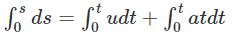= ut + 1/2at2

Derivation of Third Equation of Motion

Derivation of Third Equation of Motion by Algebraic Method

We know that, displacement is the rate of change of position of an object. Mathematically, this can be represented as:

Displacement =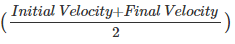× t
Substituting the standard notations, the above equation becomes
s =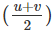× t

From the first equation of motion, we know that
v = u + at
Rearranging the above formula, we get
t = v − u/a

Substituting the value of t in the displacement formula, we get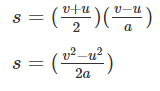2as = v− u2

Rearranging, we get
v= u+ 2as

Derivation of Third Equation of Motion by Graphical Method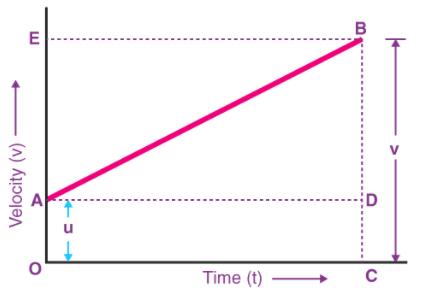From the graph, we can say that
The total distance travelled, s is given by the Area of trapezium OABC.
Hence,
S = ½ (Sum of Parallel Sides) × Height
S = (OA+CB) × OC

Since, OA = u, CB = v, and OC = t
The above equation becomes
S= ½ (u+v) × t
Now, since t = (v – u)/ a
The above equation can be written as:
S= ½ ((u+v) × (v-u))/a
Rearranging the equation, we get
S= ½ (v+u) × (v-u)/a
S = (v- u2)/2a
Third equation of motion is obtained by solving the above equation:
v2 = u+ 2aS

Derivation of Third Equation of Motion by Calculus Method

We know that acceleration is the rate of change of velocity and can be represented as:
a = dv/dt (1)
We also know that velocity is the rate of change of displacement and can be represented as:
v = ds/dt (2)

Cross multiplying (1) and (2), we get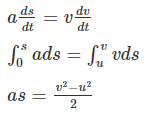v= u+ 2as

Solved Examples:

Q. An automobile traveling with a speed of 60 Km/h can apply brake to stop within a distance of 20 m If the car is going twice as fast i.e., 120 Km/h the stopping distance will be
A. 60m
B. 40m
C. 20m
D. 80m

Q2. A cart that is free to move in one dimension is moving in the positive direction and slowing down under the influence of a constant backward pull until the cart comes to a momentary stop and reverses direction. What are the signs of the cart’s velocity and acceleration during the moment it is stopped?
A. velocity : 0 acceleration : +
B. acceleration : 0 velocity : +
C. velocity : 0 acceleration : –
Answer: C. Since the cart has stopped at the moment, the speed and velocity of the cart are zero.Hence the cart achieves negative velocity which means that it should be accelerating in the negative direction to raise velocity from zero to finite value in the negative direction.

Motion Under Gravity (Free Fall)

Acceleration produced in a body by the force of gravity, is called acceleration due to gravity. It is represented by the symbol g.

Value of g=9.8 m/s= 980 cm/s2 = 32 ft/s2
In the absence of air, it is found that all bodies (irrespective of the size, weight or composition) fall with the same acceleration near the surface of the earth. This motion of a body falling towards the earth from a small altitude (h << earth's radius) is called motion under gravity. Free fall means acceleration of body is equal to acceleration due to gravity.

If a Body is Projected Vertically Upward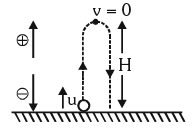Positive / Negative directions are a matter of choice. You may take another choice.

(i) Equations of motion: Taking initial position as origin and direction of motion (i.e. vertically up) as positive a = – g  [As acceleration is downwards while motion upwards]
So, if a body is projected with velocity u and after time t it reaches a height h then

v = u - gt, h = ut - 1/2gt2
v2 = u2 - 2gh, hnth = u - g/2(2n - 1)

(ii) For maximum height v = 0
So from above equation u = g t
it is called time of ascent (t1) = u/g
In case of motion under gravity, time taken to go up is equal to the time taken to fall down through the same distance. Time of descent (t2) = time of ascent (t1) = u/g

∴ Total time of flight T = t1 + t2 = 2u/g
and u2 = 2gh ⇒ H = u2/2g

If a Body is Projected Vertically Downward With Some Initial Velocity From Some Height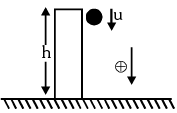Equations of motion: Taking initial position as origin and direction of motion (i.e., downward direction) as a positive, we have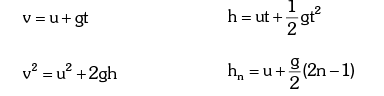The document Kinematic Equations for Uniformly Accelerated Motion - Notes | Study Physics Class 11 - NEET is a part of the NEET Course Physics Class 11.
All you need of NEET at this link: NEET

## Physics Class 11

127 videos|464 docs|210 tests

## Physics Class 11

127 videos|464 docs|210 tests

### How to Prepare for NEET

Read our guide to prepare for NEET which is created by Toppers & the best Teachers

Track your progress, build streaks, highlight & save important lessons and more!

,

,

,

,

,

,

,

,

,

,

,

,

,

,

,

,

,

,

,

,

,

;